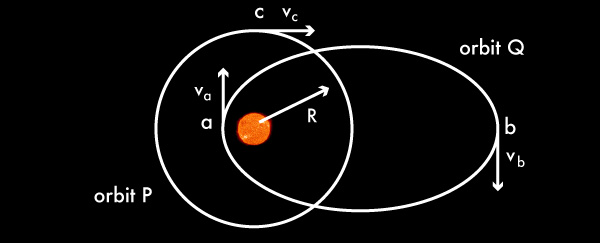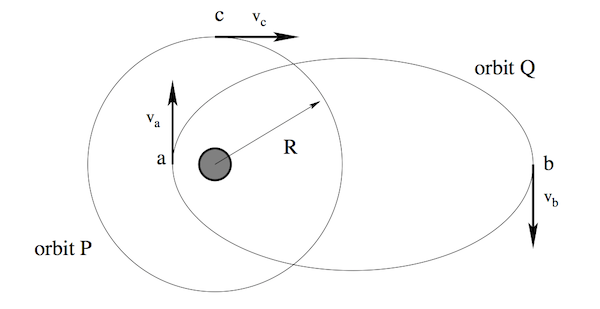# Orbiting RankingConsider the two orbits around the sun shown below. Orbit $P$ is circular with radius $R$, orbit $Q$ is elliptical such that the farthest point b is between $2R$ and $3R$, and the nearest point a is between $R/3$ and $R/2$. Consider the magnitudes of the velocity of the circular orbit $v_c$, the velocity of the comet in the elliptical orbit at the farthest point $v_b$, and the velocity of the comet in the elliptical orbit at the nearest point $v_a$. Which of the following rankings is correct?Note: This problem appeared on the 2012 F = ma exam.

×